Students can Download Tamil Nadu 12th Computer Science Model Question Paper 3 English Medium Pdf, Tamil Nadu 12th Computer Science Model Question Papers helps you to revise the complete Tamilnadu State Board New Syllabus, helps students complete homework assignments and to score high marks in board exams.

## TN State Board 12th Computer Sceince Model Question Paper 3 English Medium

General Instructions:

1. The question paper comprises of four parts.
2. You are to attempt all the parts. An internal choice of questions is provided wherever applicable.
3. All questions of Part I, II, III and IV are to be attempted separately.
4. Question numbers 1 to 15 in Part I are Multiple Choice Questions of one mark each.
These are to be answered by choosing the most suitable answer from the given four alternatives and writing the option code and the corresponding answer
5. Question numbers 16 to 24 in Part II are two-mark questions. These are to be answered in about one or two sentences.
6. Question numbers 25 to 33 in Part III are three-mark questions. These are to be answered in about three to five short sentences.
7. Question numbers 34 to 38 in Part IV are five-mark questions. These are to be answered in detail Draw diagrams wherever necessary.

Time: 3 Hours
Maximum Marks: 70

PART – 1

Choose the correct answer. Answer all the questions: [15 × 1 = 15]

Question 1.
Sin (0) = 0 is an example for function.
(a) Impure
(b) Pure
(c) Interface
(d) Instruction
(b) PureQuestion 2.
Write the output (value stored in b)
1. a:=5
2. b:=a
(a) 0
(b) 3
(c) 5
(d) 2
(c) 5

Question 3.
Which of the following shortcut is used to create new Python Program?
(a) Ctrl + C
(b) Ctrl + F
(c) Ctrl + B
(d) Ctrl + N
(d) Ctrl + NQuestion 4.
Escape sequences can be given using ……………………. parameter in print( ) function.
(a) Ret
(b) Let
(c) End
(d) Sep
(c) End

Question 5.
Pick the correct one to execute the given statement successfully,
if_: print(x,” is a leap year”)
(a) x % 2 = 0
(b) x % 4 = = 0
(c) x/4 = 0
(d) x % 4 = 0
(b) x % 4 = = 0Question 6.
Find the wrong statement from the following.
(i) Slice a single character from a string
(ii) Slice a substring
(iii) Slice a substring without specifying beginning index
(iv) Slice a substring without specifying end index
(a) (i), (ii)
(b) (ii), (iii), (iv)
(c) All are wrong
(d) All are correct
(d) All are correct

Question 7.
Write the output.
list = [34, 45, 48]
list.append(90)
(a) [34, 45, 48, 90]
(b) [90, 34, 45, 48]
(c) [34, 90, 45, 48]
(d) [34, 45, 90, 48]
(a) [34, 45, 48, 90]Question 8.
Match the following.
1. constructor – (I) def process(self)
2. Destructor – (II) S.x
3. method – (III) _del_(self)
4. object – (IV) _init_(self, num)
(a) 1-(IV) 2-(III) 3-(I) 4-(II)
(b) 1 -(I) 2-(II) 3-(III) 4-(IV)
(c) 1-(IV) 2-(II) 3-(I) 4-(III)
(d) 1 -(I) 2-(III) 3-(IV) 4-(II)
(a) 1-(IV) 2-(III) 3-(I) 4-(II)

Question 9.
Match the following
1. Relational – (I) Classes
2. Object model – (II) Mainframe
3. ER model – (III) Key
4. Hierarchical – (IV) Entity
(a) 1-(III), 2-(I), 3-(IV), 4-(II)
(b) 1-(I), 2-(II), 3-(III), 4-(IV)
(c) 1-(IV), 2-(III), 3-(I), 4-(II)
(d) 1-(IV), 2-(II), 3-(I), 4-(III)
(a) 1-(III), 2-(I), 3-(IV), 4-(II)Question 10.
Identify which statement is given wrongly?
(a) DDL statement should specify the proper data type
(b) DDL should not identify the type of data division
(c) DDL may define the range of values
(d) DDL should define the size of the data item
(b) DDL should not identify the type of data division

Question 11.
(I) csv.writer work with list/tuple
(II) csv.Dictwriter work with dictionary
(a) (I), (II) – True (III) – False
(b) (I) -True (II), (III) – False
(c) (I), (II), (III) – True
(d) (I), (II), (III) – False
(a) (I), (II) – True (III) – FalseQuestion 12.
Pick the odd one out.
(a) Perl
(b) Ruby
(c) Tel
(d) Java
(d) Java

Question 13.
Match the following related to OS module.
1. g++ – (I) Name of C++ file
2. variable namel – (II) General compiler
3. mode – (III) Input output mode
4. variable_name2 – (IV) Name of exe file
(a) 1-(I), 2-(II), 3-(III), 4-(IV)
(b) 1-(II), 2-(I), 3-(III), 4-(IV)
(c) 1-(IV), 2-(III), 3-(II), 4-(I)
(d) 1-(IV), 2-(I), 3-(III) , 4-(II)
(b) 1-(II), 2-(I), 3-(III), 4-(IV)Question 14.
Assertion (A): Query result can be stored in csv file.
Reason (R): To display the query output in a tabular form.
(a) R is the reason for A
(b) R is wrong
(c) A is wrong
(d) Both are not related
(a) R is the reason for A

Question 15.
Identify the Incorrect pair
1. Group – (i) Aggregate functions
2. Order by – (ii) Sortind data
3. Having – (iii) Filter data
4. Where – (iv) max, min
(a) (2)
(b) (1)
(c) (4)
(d) (3)
(c) (4)

PART – II

Answer any six questions. Question No. 21 is compulsory. [6 × 2 = 12]

Question 16.
Write note on keyword argument?
Keyword arguments will invoke the function after the parameters are recognized by their parameter names. The value of the keyword argument is matched with the parameter name and so, one can also put arguments in improper order (not in order).Question 17.
What is composition in functions?
The value returned by a function may be used as an argument for another function in a nested manner. This is called composition. For example, if we wish to take a numeric value or an expression as a input from the user, we take the input string from the user using the function input( ) and apply eval( ) function to evaluate its value.

Question 18.
Write note on len (str)?
Syntax : len(str)
Description: Returns the length (no of characters) of the string.
Example: >>> A=”Corporation”
>>> print(len(A))
11Question 19.
Define sequence data type?
Python programming language has four collections of data types such as List, Tuples, Set – and Dictionary. A list in Python is known as a “sequence data type” like strings. It is an ordered collection of values enclosed within square brackets [ ].

Question 20.
Give example for modifying variable outside a function?
Example:
let y: = 0
(int) inc (int) x
y: = y + x;
return (y)

Question 21.
Abstract Data type (ADT) is a type (or class) for objects whose behavior is defined by a set of value and a set of operations.

Question 22.
Write note on Boolean data type?
A Boolean data can have any of the two values: True or False.
Example :
Bool_varl=True
Bool_var2=FalseQuestion 23.
Write note on column, table constraint?
Column constraint: Column constraint apply only to individual column.
Table constraint: Table constraint apply to a group of one or more columns.

Question 24.
What are the two ways of passing arguments in variable length method?

1. Non keyword variable arguments
2. Keyword variable arguments

PART – III

Answer any six questions. Question No. 29 is compulsory. [6 × 3 = 18]

Question 25.
Explain print ( ) in python?
In Python, the print( ) function is used to display result on the screen. The syntax for print( ) is as follows:
Example:
print (“string to be displayed as output ”) print (variable)
print (“String to be displayed as output ”, variable)
print (“Stringl ”, variable, “String 2”, variable, “String 3” )Question 26.
Give the rules for variables?
An Identifier is a name used to identify a variable, function, class, module or object.

1. An identifier must start with an alphabet (A..Z or a..z) or underscore (_).
2. Identifiers may contain digits (0 .. 9)
3. Python identifiers are case sensitive, i.e., uppercase and lowercase letters are distinct.
4. Identifiers must not be a python keyword.
5. Python does not allow punctuation character such as %,\$, @ etc., within identifiers.

Question 27.
Explain commands and their functions in SQL?
Tables are the only way to store data, therefore all the information has to be arranged in the form of tables. The SQL provides a predetermined set of commands to work on databases.

Keywords:
They have a special meaning in SQL. They are understood as instructions.

Commands:
They are instructions given by the user to the database also known as statements.

Clauses:
They begin with a keyword and consist of keyword and argument.

Arguments:
They are the values given to make the clause complete.Question 28.
Write note on abs ( ), Ord ( ), Chr ( )?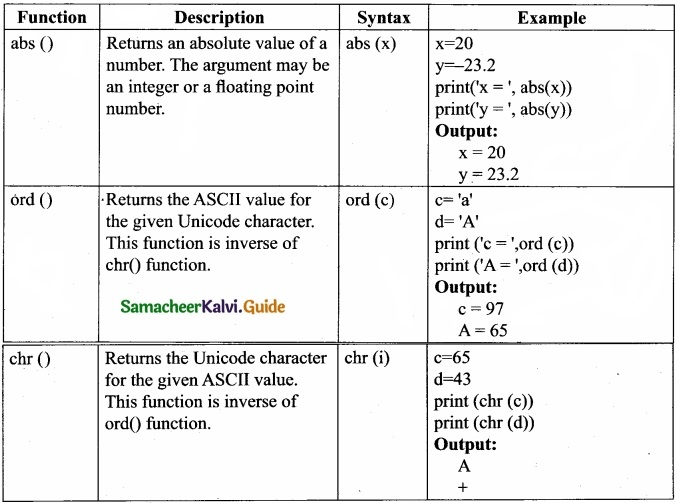Question 29.
Write a pure function to find Gcd of 2 numbers?
let rec gcd a b :=
if b < > 0 then gcd b (a mod b) else return a;;
output
gcd 13 27;;
-: int = 1
gcd 20536 7826;;
-: int = 2
In the above example program ‘gcd’ is the name of the function which recursively called till the variable ‘b’ becomes ‘O’. Remember b and (a mod b) are two arguments passed to ‘a’ and ‘b’of the gcd function.Question 30.
Write the use of Savepoint command with an example?
SAVEPOINT command:
The SAVEPOINT command is used to temporarily save a transaction so that you can rollback to the point whenever required. The different states of our table can be saved at anytime using different names and the rollback to that state can be done using the ROLLBACK command.

SAVEPOINT savepoint name;
UPDATE Student SET Name = ‘Mini’WHERE Admno=105;
SAVEPOINT A;

Question 31.
Find output:
str1 = input (“Enter a string: “)
index= -1
while index >= -(len(str1)):
print (“Subscriptf”,index,”] : ” + str1 [index])
index += -1
Output:
Enter a string: welcome
Subscript [ -1 ] : e
Subscript [ -2 ] : m
Subscript [ -3 ] : o
Subscript [ -4] : c
Subscript [ -5 ] : 1
Subscript [ -6 ] : e
Subscript [ -7 ] : wQuestion 32.
Name some functions where lambda 0 is used?
filter ( ), map ( ), reduce

Question 33.
Find output:
def
printinfo (name, sal=500):
print (name)
print (sal)
return
print info (“mani”)
Output:
Mani
500

PART – IV

Answer all the questions. [5 × 5 = 25]

Question 34 (a).
Explain, various data types in SQL?[OR]

(b) Compare DBMS with RDBMS?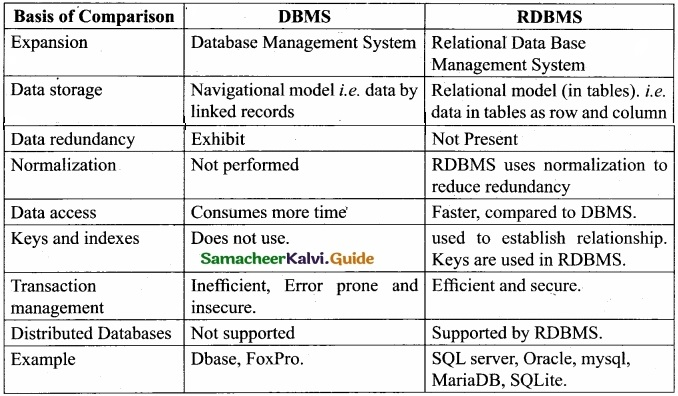Question 35 (a).
Explain various characteristics of modules?

1. Modules contain instructions, processing logic, and data.
2. Modules can be separately compiled and stored in a library.
3. Modules can be included in a program.
4. Module segments can be used by invoking a name and some parameters.
5. Module segments can be used by other modules.

[OR]

(b) Explain Alter command in SQL?
The ALTER command is used to alter the table structure like adding a column, renaming the existing column, change the data type of any column or size of the column or delete the column from the table. It is used in the following way:

ALTER TABLE <table-name> ADD <column-name> <data type> <size>;
To add a new column “Address” of type ‘char’ to the Student table, the command is used as:-

To modify existing column of table, the ALTER TABLE command can be used with MODIFY clause like wise:

ALTER <table-name> MODIFY<column-name> <data type> <size>;
ALTER TABLE Student MODIFY Address char (25);

The above command will modify the address column of the Student table to now hold 25 characters. The ALTER command can be used to rename an existing column in the following way:

ALTER <table-name> RENAME old-column-name TO new-column-name;
For example to rename the column Address to City, the command is used as:

ALTER TABLE Student RENAME Address TO City;
The ALTER command can also be used to remove a column or all columns, for example to remove a particular column, the DROP COLUMN is used with the ALTER TABLE to remove a particular field, the command can be used as:

ALTER <table-name> DROP COLUMN <column-name>;
To remove the column City from the Student table, the command is used as:
ALTER TABLE Student DROP COLUMN City;Question 36(a).
Consider the following employee table. Write SQL commands for the qtns.(i) to (v)?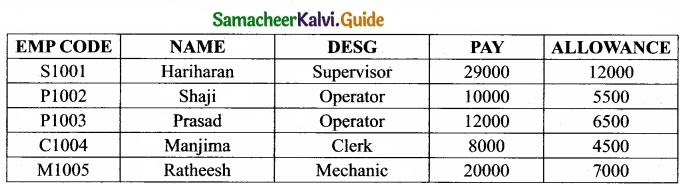1. To display the details of all employees in descending order of pay.
2. To display all employees whose allowance is between 5000 and 7000.
3. To remove the employees who are mechanic.
4. To add a new row.
5. To display the details of all employees who are operators.

Output:

1. SELECT * FROM employee ORDER BY DESC;
2. SELECT * FROM employee WHERE ((allowance >= 5000) AND (allowance <=7000));
3. DELETE FROM employee WHERE desig = “Mechanic”;
4. INSERT INTO employee(Empcode, Name, desig, pay, allowance) VALUES(‘M1006’, ‘RAM’, ‘Mechanic’,22000, 8000);
5. SELECT * FROM employee WHERE desig = ‘operator’;

[OR]

(b) How will you write data into different types in CSV files?
As you know Python provides an easy way to work with CSV file and has csv module to read and write data in the csv file. In the previous topics, You have learned how to read CSV files • in Python. In similar way, You can also write a new or edit an existing CSV files in Python.

1. Creating A New Normal CSV File
2. Modifying An Existing File
3. Writing On A CSV File with Quotes
4. Writing On A CSV File with Custom Delimiters
5. Writing On A CSV File with Lineterminator
6. Writing On A CSV File with Quotechars
7. Writing CSV File Into A Dictionary
8. Getting Data At Runtime And Writing In a FileQuestion 37 (a).
Write a sample program to illustrate private and public variables using classes?
class Sample:
def_init_(self, n1, n2):
self._n1=n1
self._n2=n2
def display(self):
print(“Class variable 1 = “, self.n1)
print(“Class variable 2 = “, self._n2)
S=Sample(12, 14)
S.display( )
print(“Value 1 = “, S.n1)
print(“Value 2 = “, S._n2)
In the above program, there are two class variables n1 and n2 are declared. The variable nl is a public variable and n2 is a private variable. The display( ) member method is defined to show the values passed to these two variables.

The print statements defined within class will successfully display the values of n1 and n2, even though the class variable n2 is private. Because, in this case, n2 is called by a method defined inside the class. But, when we try to access the value of n2 from outside the class Python throws an error. Because, private variable cannot be accessed from outside the class.

Output:
Class variable 1 = 12 Class variable 2=14 Value 1 = 12
Traceback (most recent call last):
File “D:/Python/Class-Test-04.py”, line 12, in <module> print(“Value 2 = “, S._n2)
AttributeError: ‘Sample’ object has no attribute ‘n2’

[OR]

(b) Explain updating and deleting Tuple with example?
As you know a tuple is immutable, the elements in a tuple cannot be changed. Instead of altering values in a tuple, joining two tuples or deleting the entire tuple is possible.
Example:
# Program to join two tuples
Tup 1 = (2, 4, 6, 8, 10)
Tup 2 = (1,3,5,7,9)
Tup 3 = Tup1 + Tup2
print(Tup3)

Output:
(2, 4, 6, 8, 10, 1, 3, 5, 7, 9)
To delete an entire tuple, the del command can be used.
Syntax: del tuple_name
Example:
Tup1 =(2, 4, 6, 8, 10)
print(“The elements of Tup1 is “, Tup1)
del Tup1
print (Tup1)

Output:
The elements of Tupl is (2, 4, 6, 8, 10)
Traceback (most recent call last):
File “D:/Python/Tuple Examp l.py”, line 4, in <module>
print (Tupl)
NameError: name ‘Tup1’ is not defined
Note that, the print statement in the above code prints the elements. Then, the del statement deletes the entire tuple. When you try to print the deleted tuple, Python shows the error.Question 38 (a).
Explain nested If -elif – else statement with example?
When we need to construct a chain of if statement(s) then ‘elif’ clause can be used instead of ‘else’.
Syntax:
if <condition-l>:
statements-block 1

elif <condition-2>:
statements-block 2

else:
statements-block n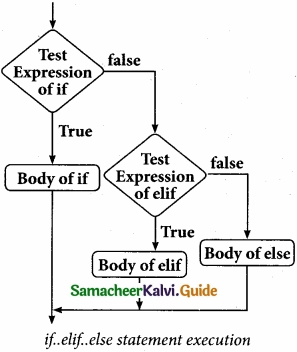In the syntax of if.elifi.else mentioned above, condition-1 is tested if it is true then statements- block 1 is executed, otherwise the control checks . condition-2, if it is true statements-block2 is executed and even if it fails statements-block n mentioned in else part is executed.

‘elif’ clause combines ifi.else-ifi.else statements to one if..elif…else, ‘elif’ can be considered to be abbreviation of ‘else if’. In an ‘if’ statement there is no limit of ‘elif’ clause that can be used, but an ‘else’ clause if used should be placed at the end.

Example:
Program to illustrate the use of nested if statement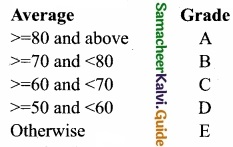m1=int (input(“Enter mark in first subject: ”))
m2=int (input(“Enter mark in second subject: ”))
avg= (m1+m2)/2
if avg>=80:
elif avg>=70 and avg<80:
elif avg>=60 and avg<70:
elif avg>=50 and avg<60:
else:

Output 1:
Enter mark in first subject: 34 Enter mark in second subject: 78 Grade : D

Output 2 :
Enter mark in first subject: 67

[OR]

(b) Explain how to pass parameters in functions?